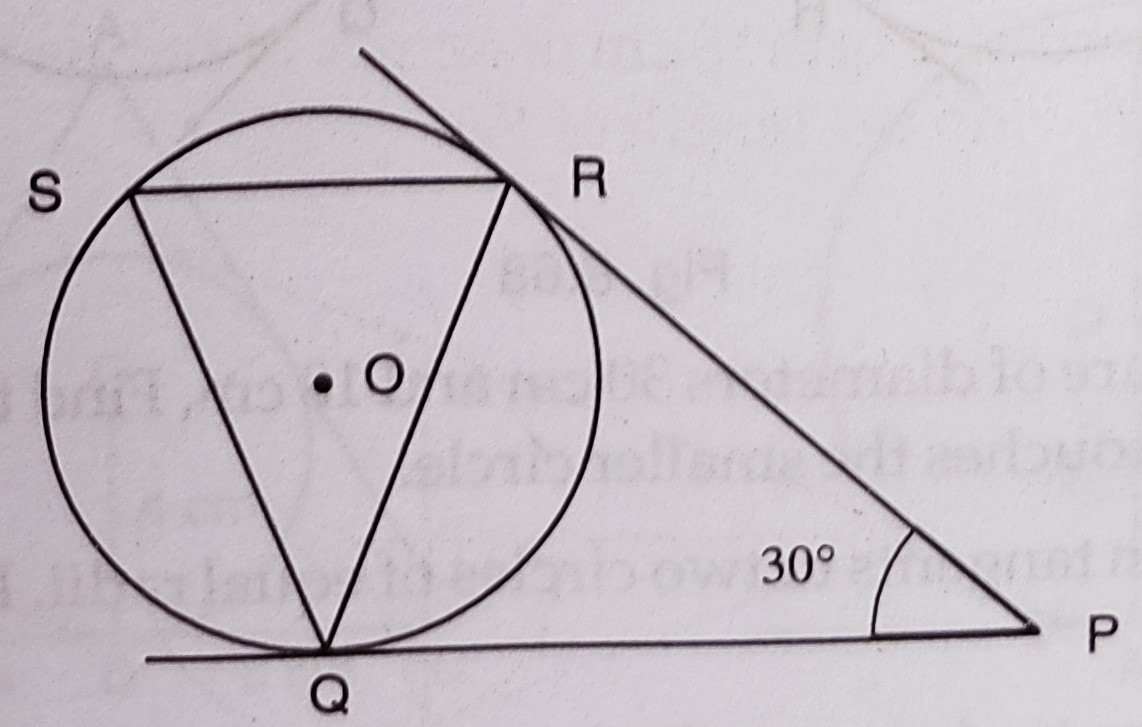# In the figure, tangents $P Q$ and $P R$ are drawn from an external point $P$ to a circle with centre $O$, such that $\angle R P Q=30^{\circ} .$ A chord $R S$ is drawn parallel to the tangent $P Q$. Find $\angle R Q S$."

Given:

In the figure, tangents $P Q$ and $P R$ are drawn from an external point $P$ to a circle with centre $O$, such that $\angle R P Q=30^{\circ} .$ A chord $R S$ is drawn parallel to the tangent $P Q$.

To do:

We have to find $\angle R Q S$.

Solution:

$PQ$ and $PR$ are tangents to the circle with centre $O$ drawn from $P$ and $\angle RPQ = 30^o$

$RS\ \parallel\ PQ$.
$PQ = PR$    (Tangents to the circle from an external point are equal)

$\angle PRQ = \angle PQR$

$\angle PRQ=\angle PQR=\frac{180^{\circ}-30^{\circ}}{2}$

$=\frac{150^{\circ}}{2}=75^{\circ}$

$\angle SRQ=\angle PQR=75^{\circ}$

$\angle RSQ=\angle QRS$      (since $QR=QS$)

$=75^{\circ}$

In $\Delta QRS$,

$\angle RQS=180^{\circ}-(\angle RSQ+\angle QRS)$

$=180^{\circ}-(75^{\circ}+75^{\circ})$

$=180^{\circ}-150^{\circ}$

$=30^{\circ}$

Therefore, $\angle RQS=30^{\circ}$.